# Telangana SSC Board (TS SSC) Question Paper 2 for Class 10th Maths 2015 In PDF

## Telangana 10th Annual Exam Question Papers 2015 Maths Paper 2 with Solutions – Free Download

Telangana SSC Class 10 Maths 2015 Question Paper 2 with solutions are available at BYJU’S in an easily downloadable pdf format, which will help the students to access at any time. Expert faculty at BYJU’S prepared solutions for all Telangana board SSC previous year question papers, by covering all the important steps, which ensure the full marks in the exam. Students can get the solutions of 2015 Class 10 Maths paper 2 in such a way, so that they can understand them easily without any confusion. Besides, images are also given for a better understanding of the solutions here. Thus, answering maths questions will improve the analytical skills of students and will help them in reaching the right approach quickly.

### Telangana Board SSC Class 10 Maths 2015 Question Paper 2 with Solutions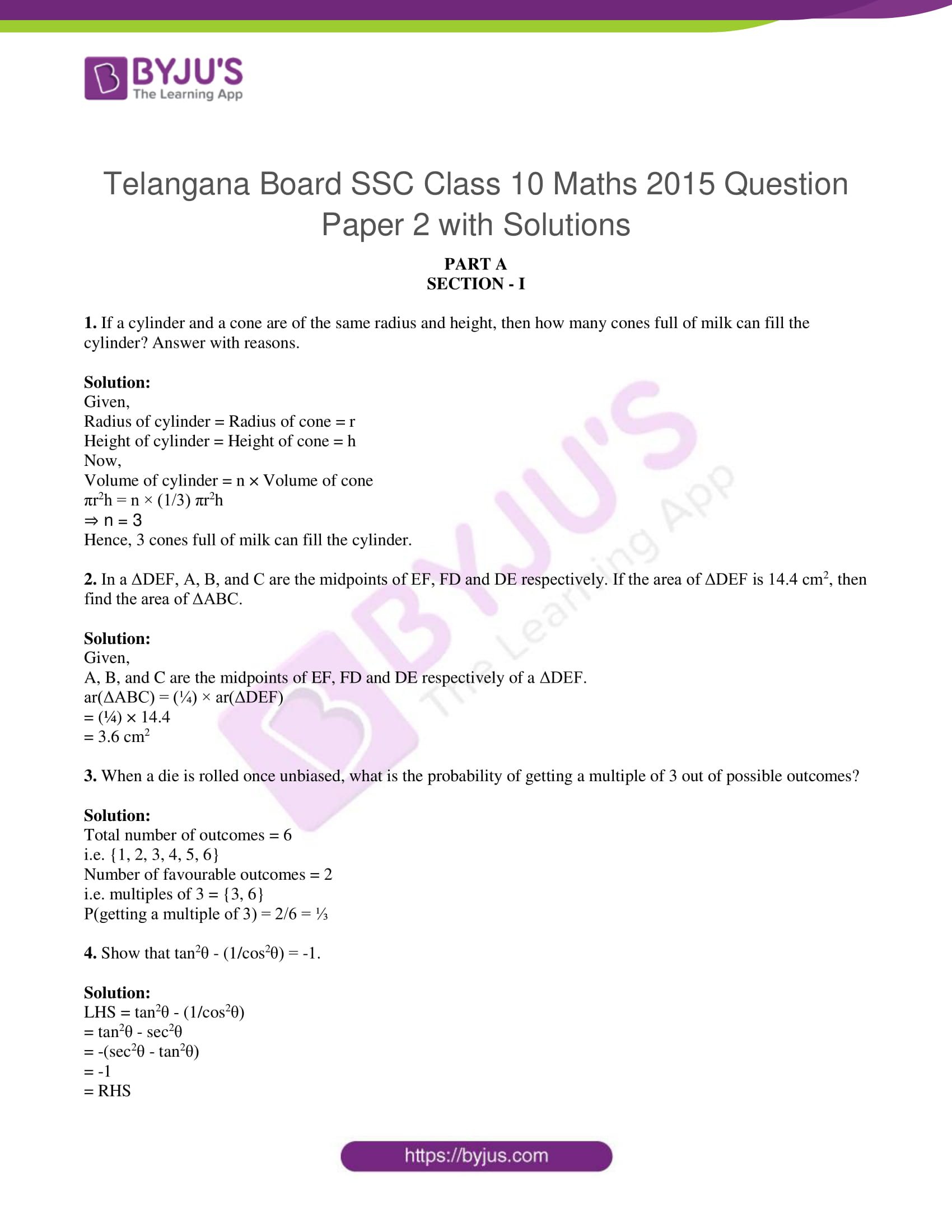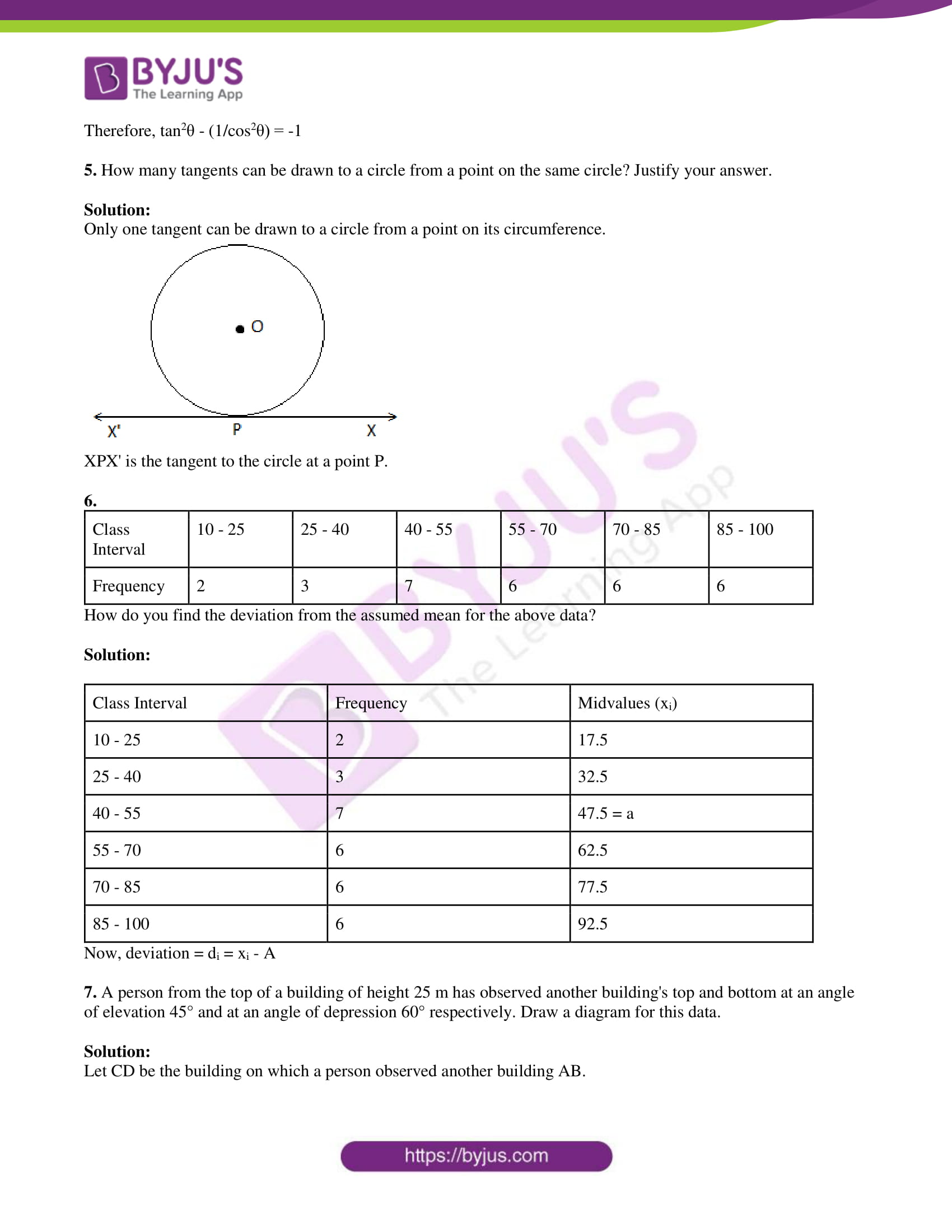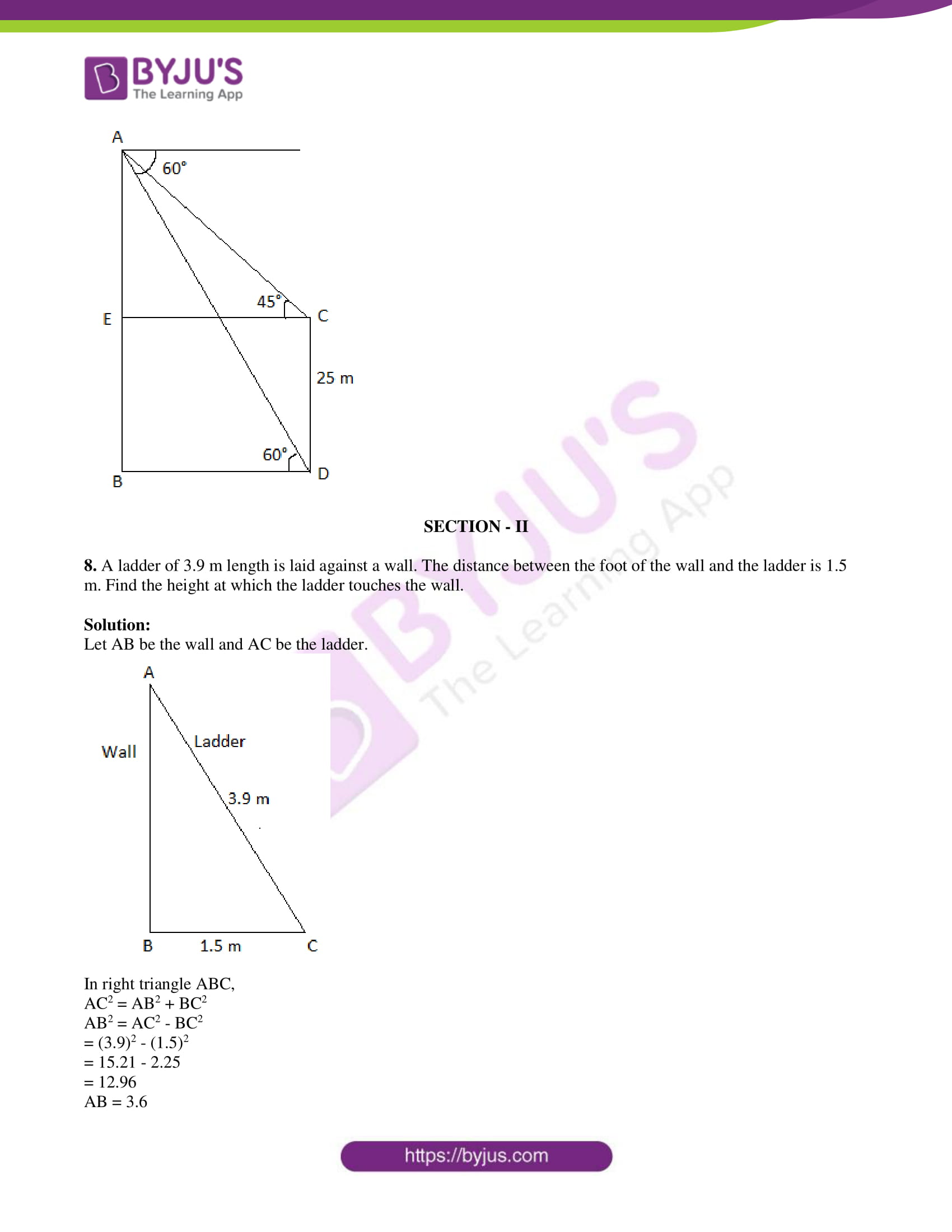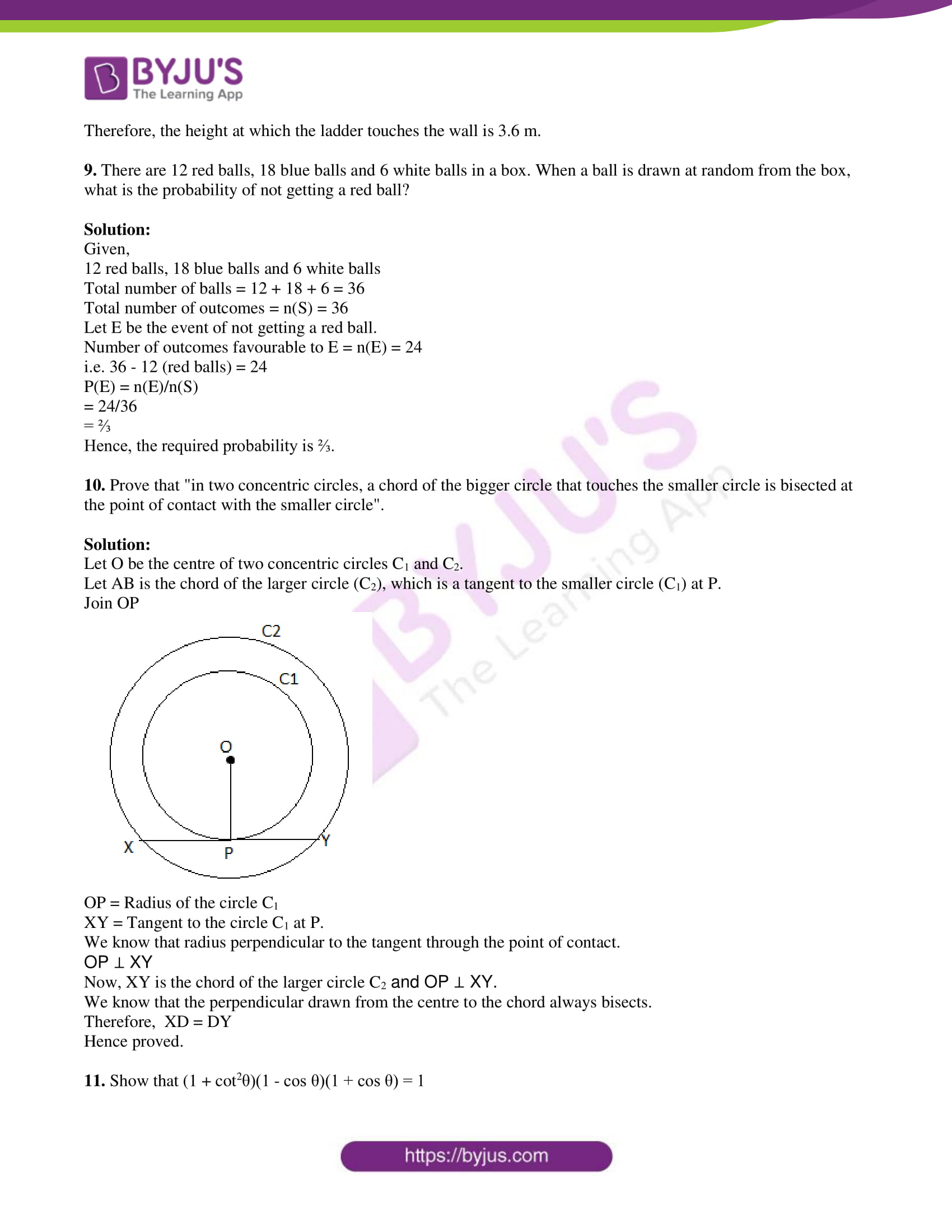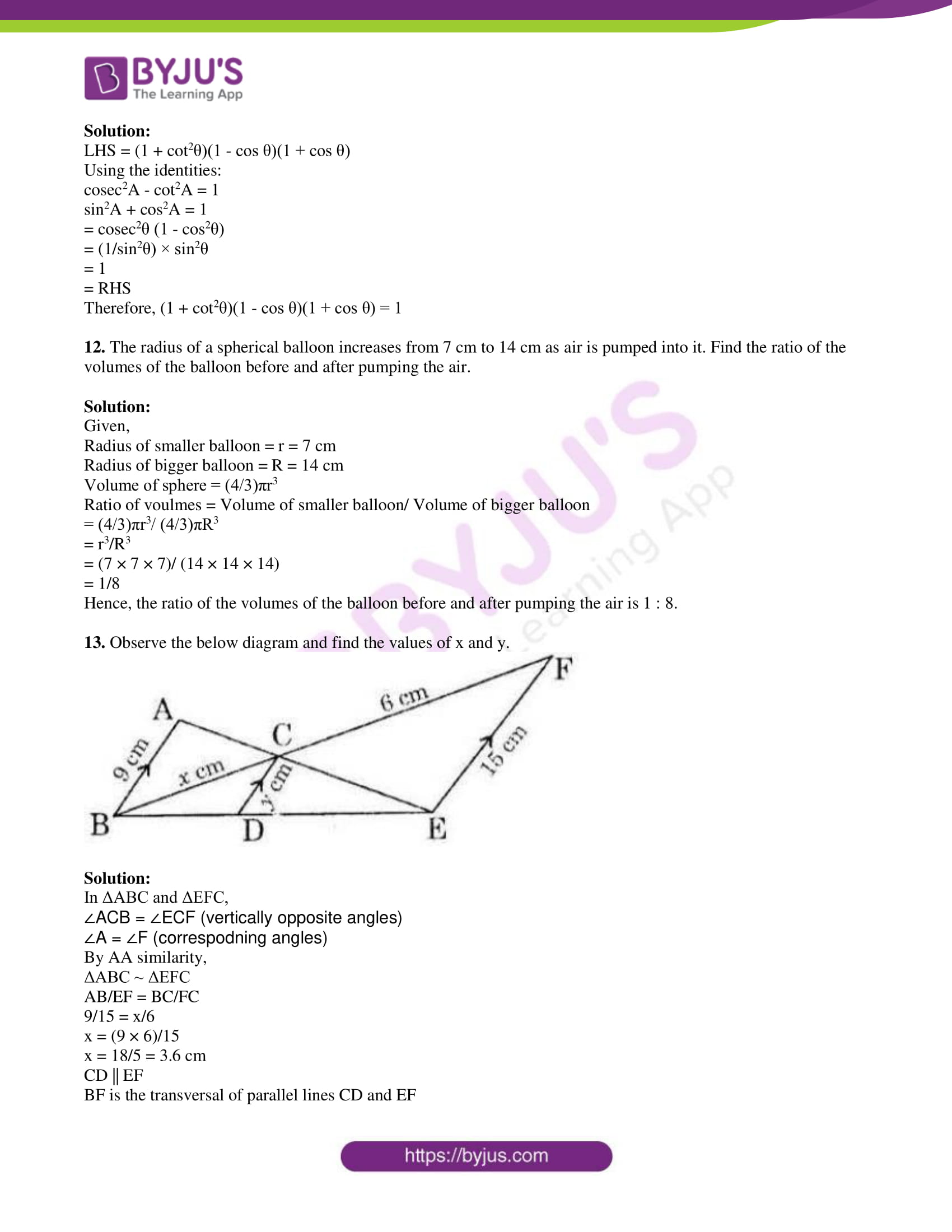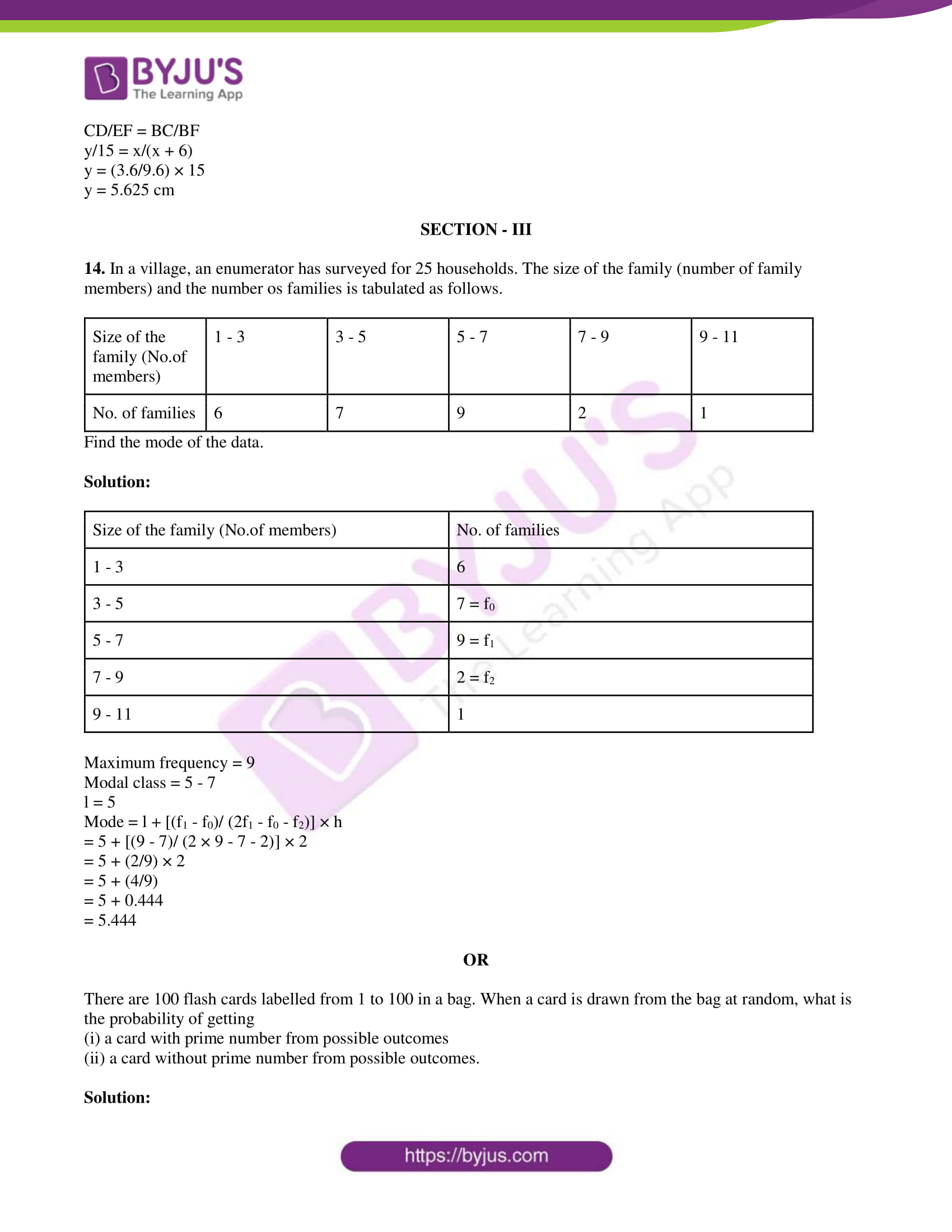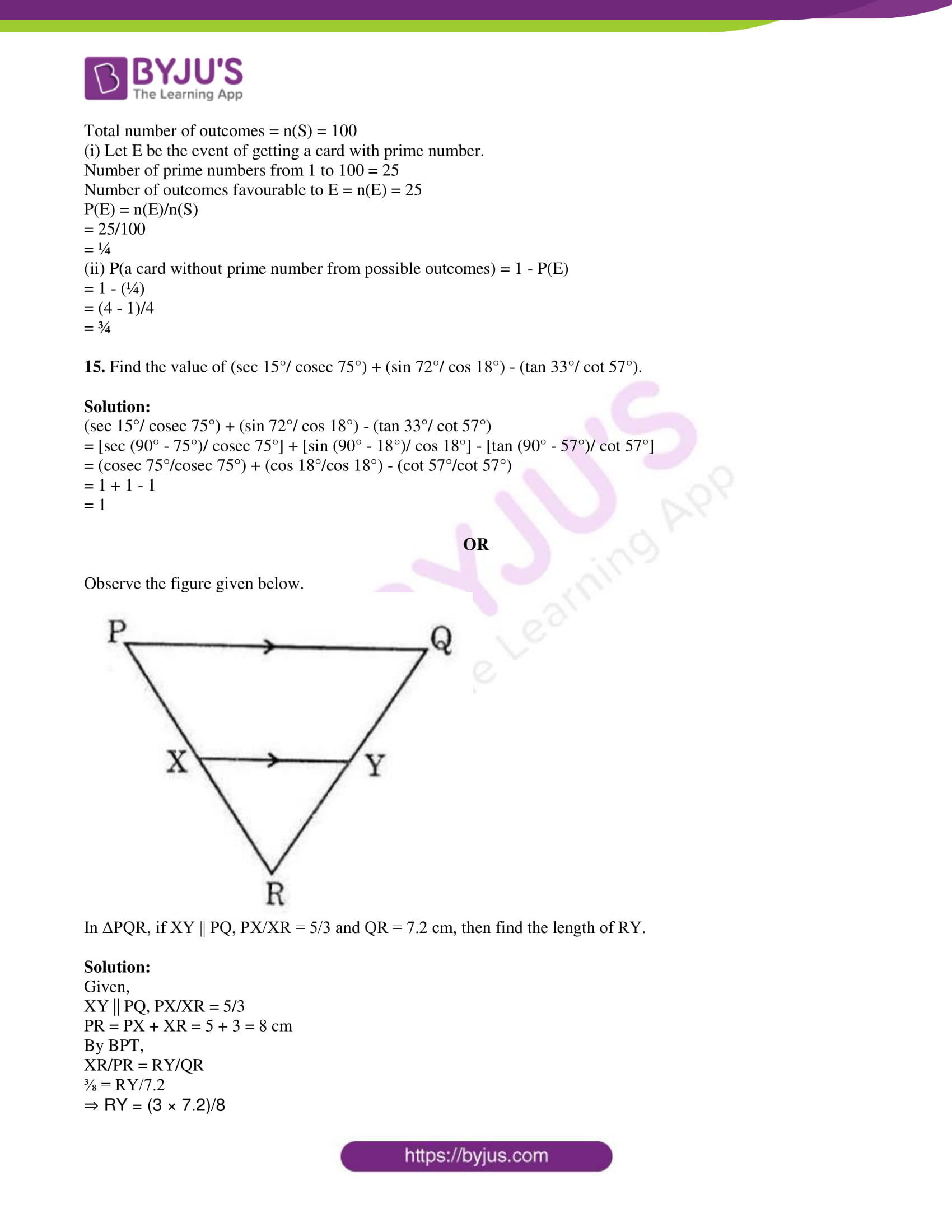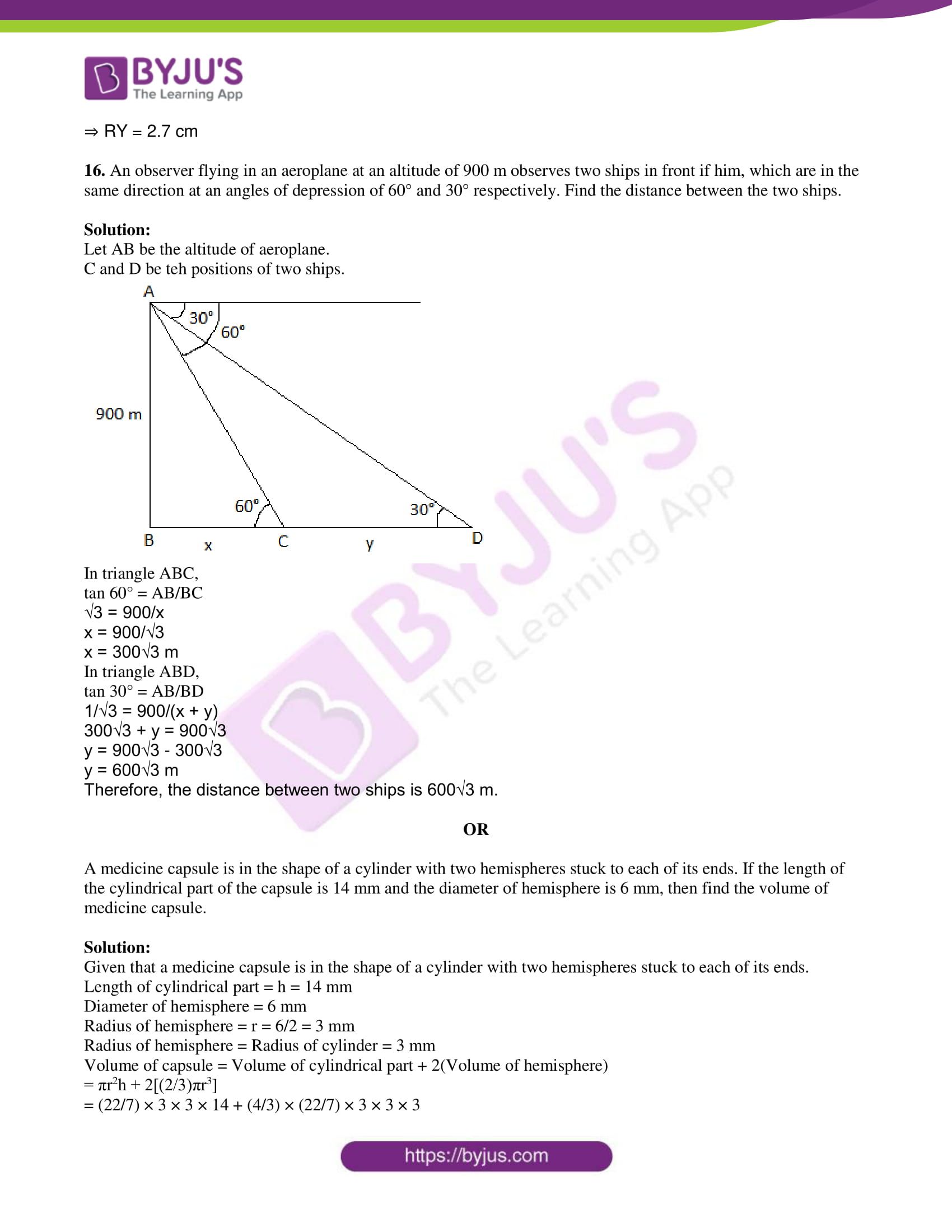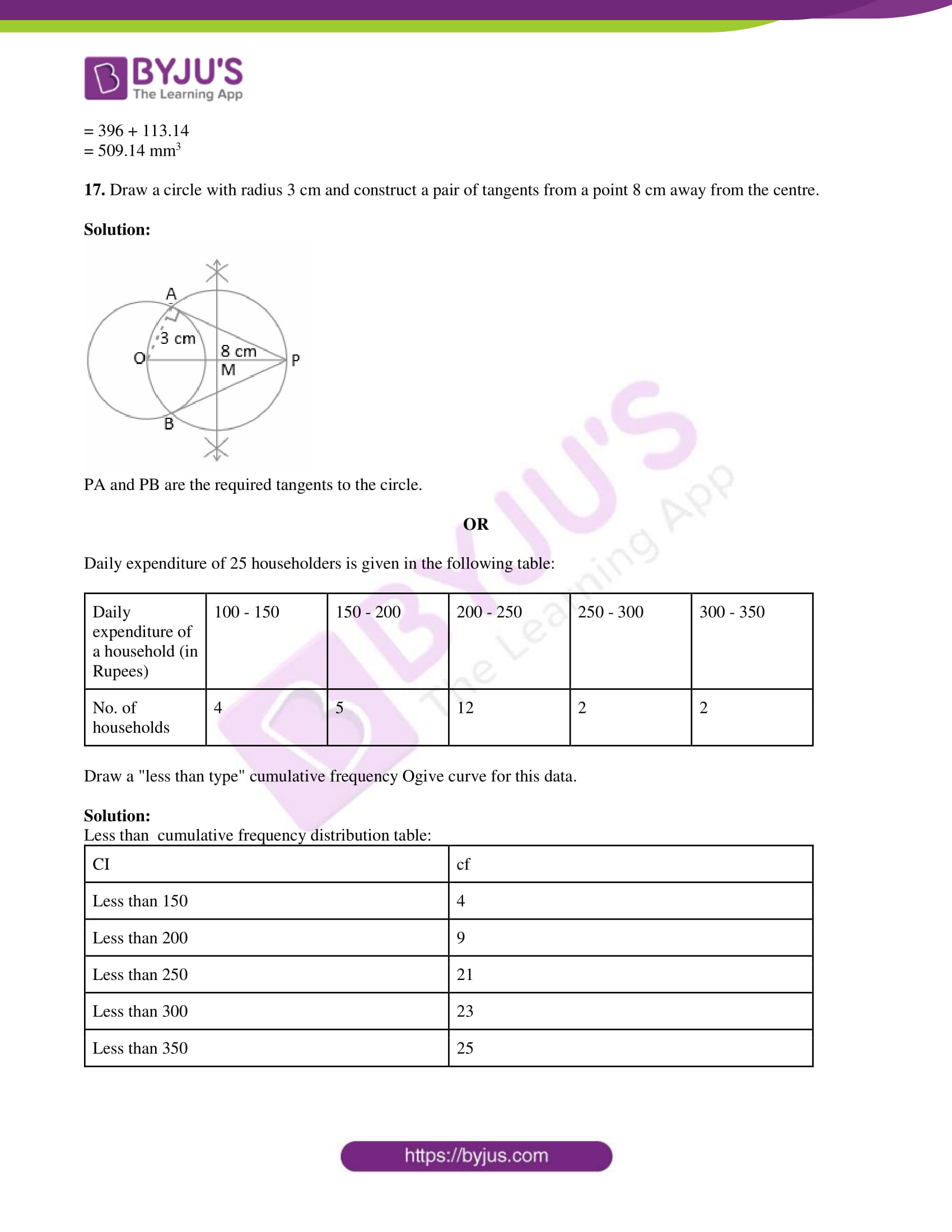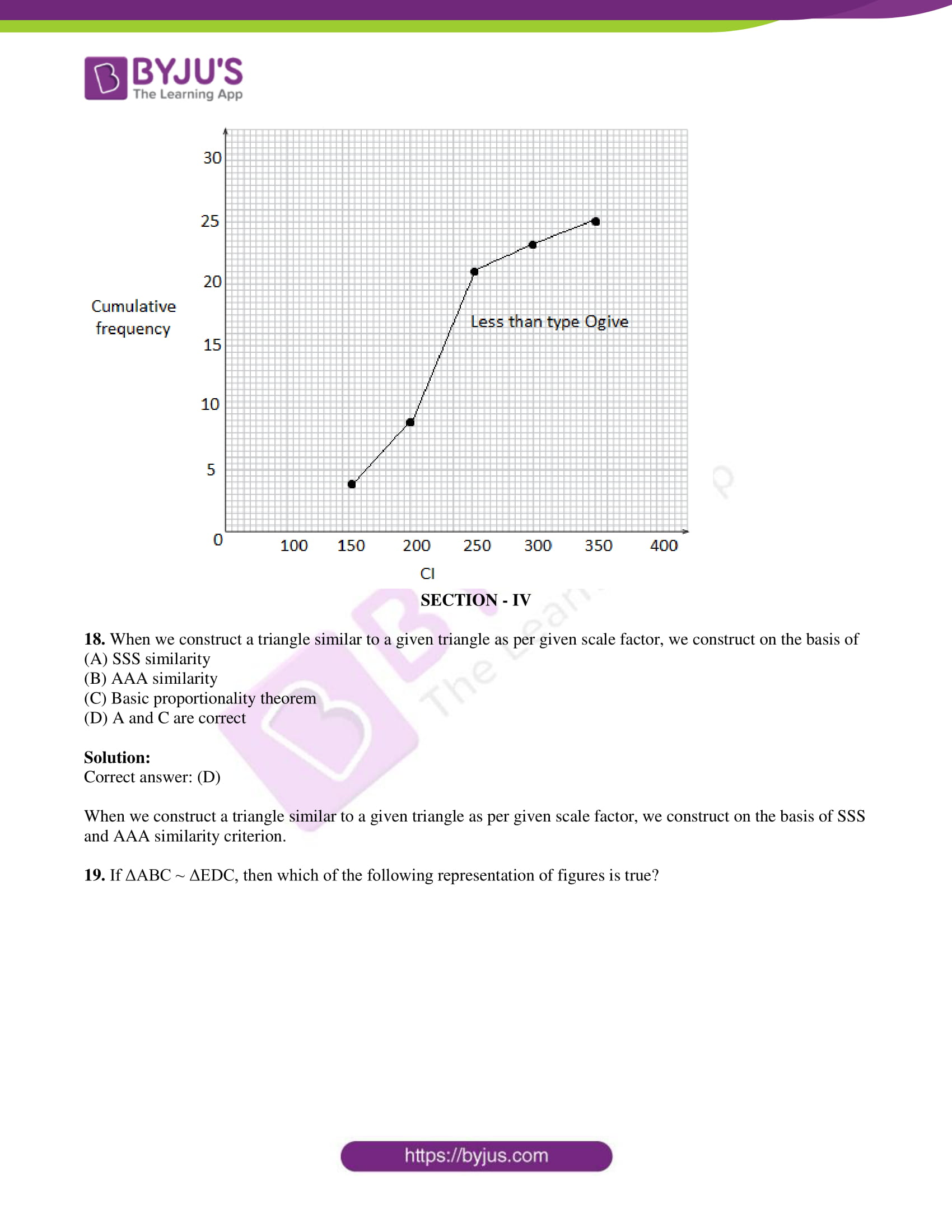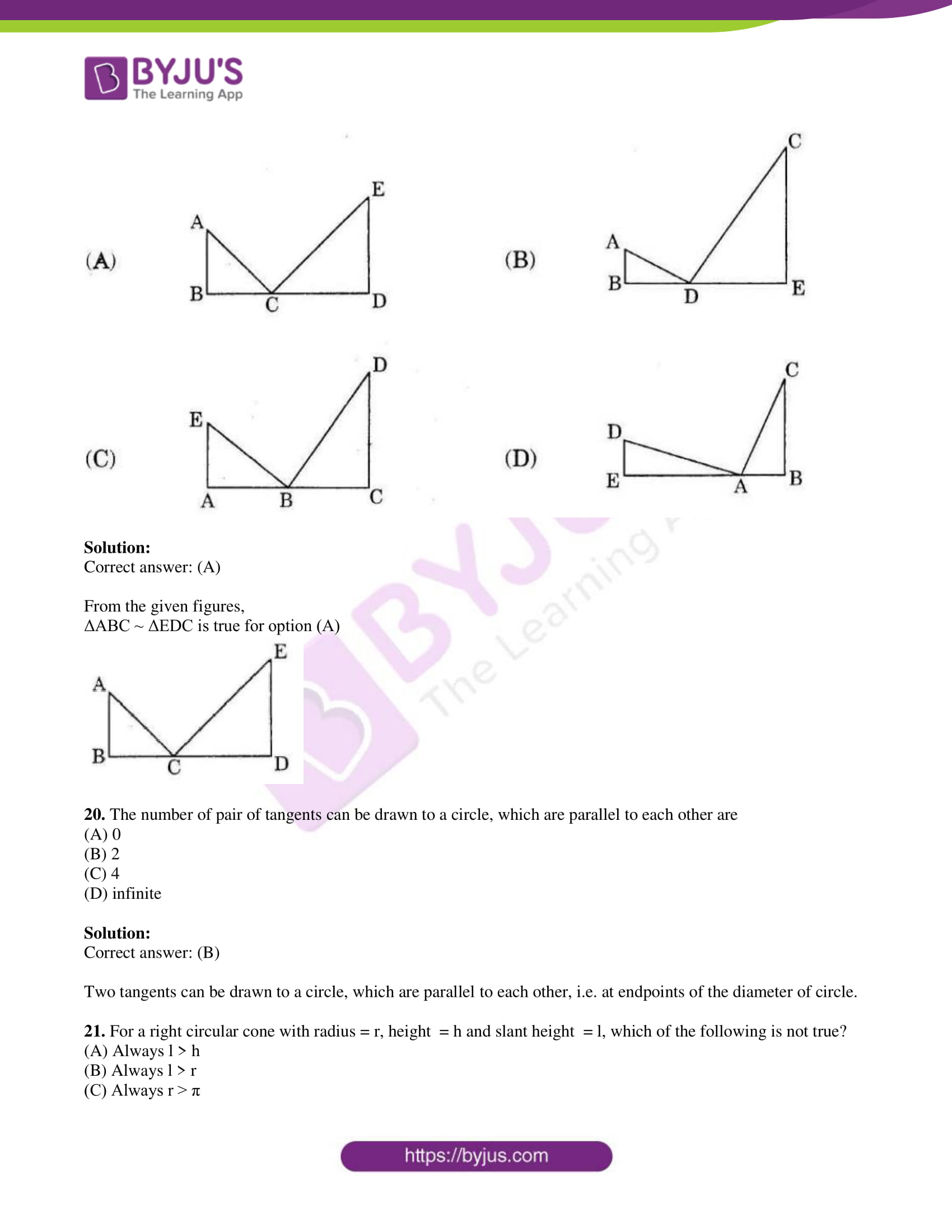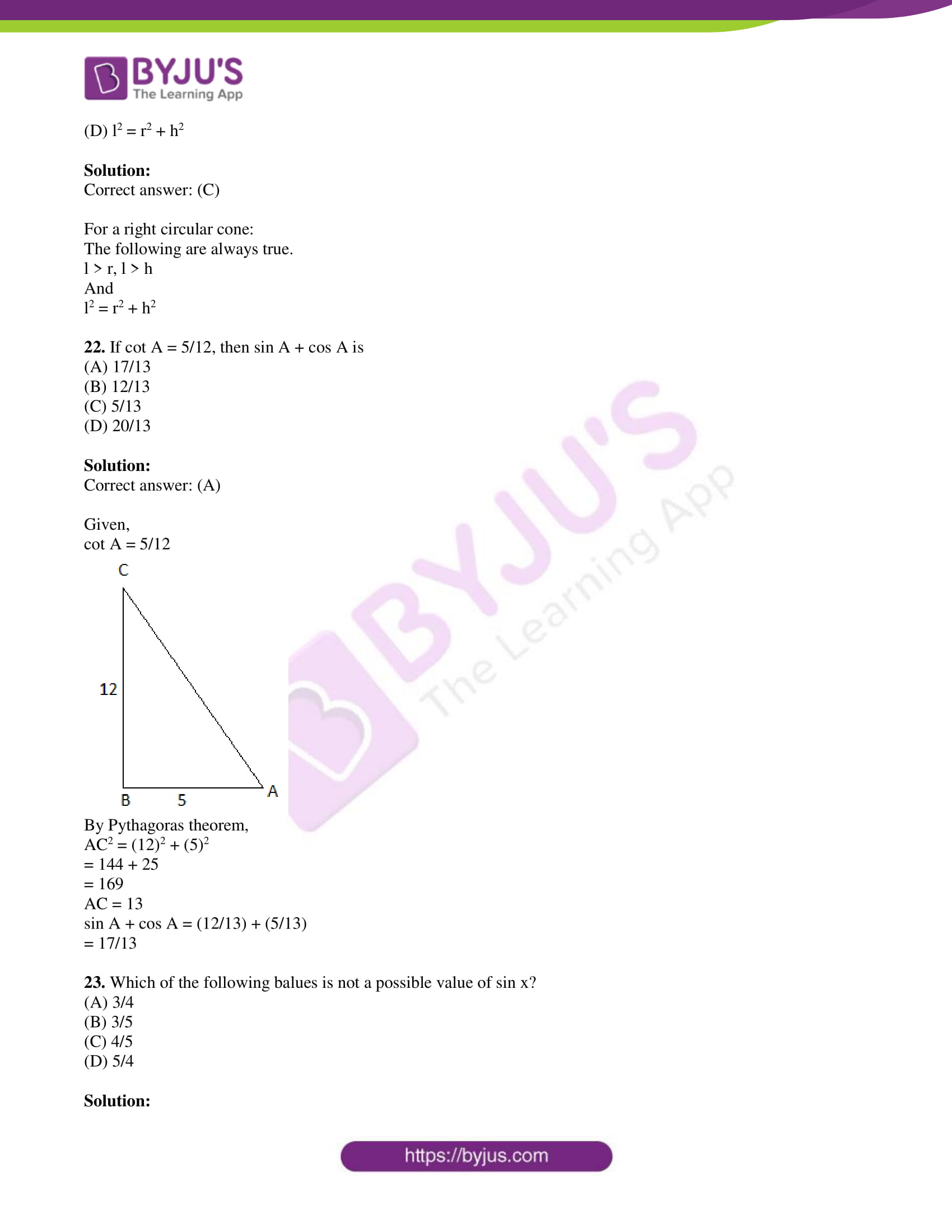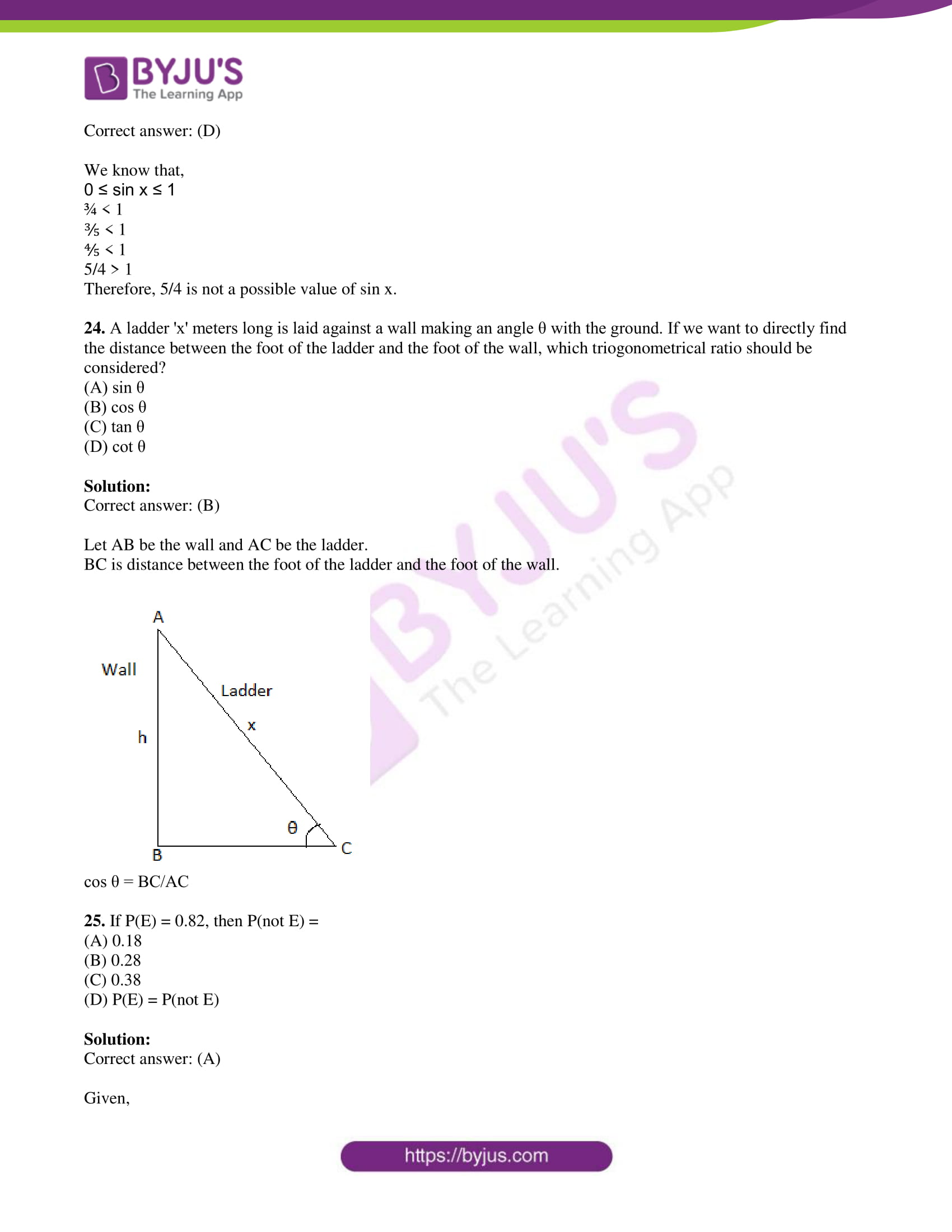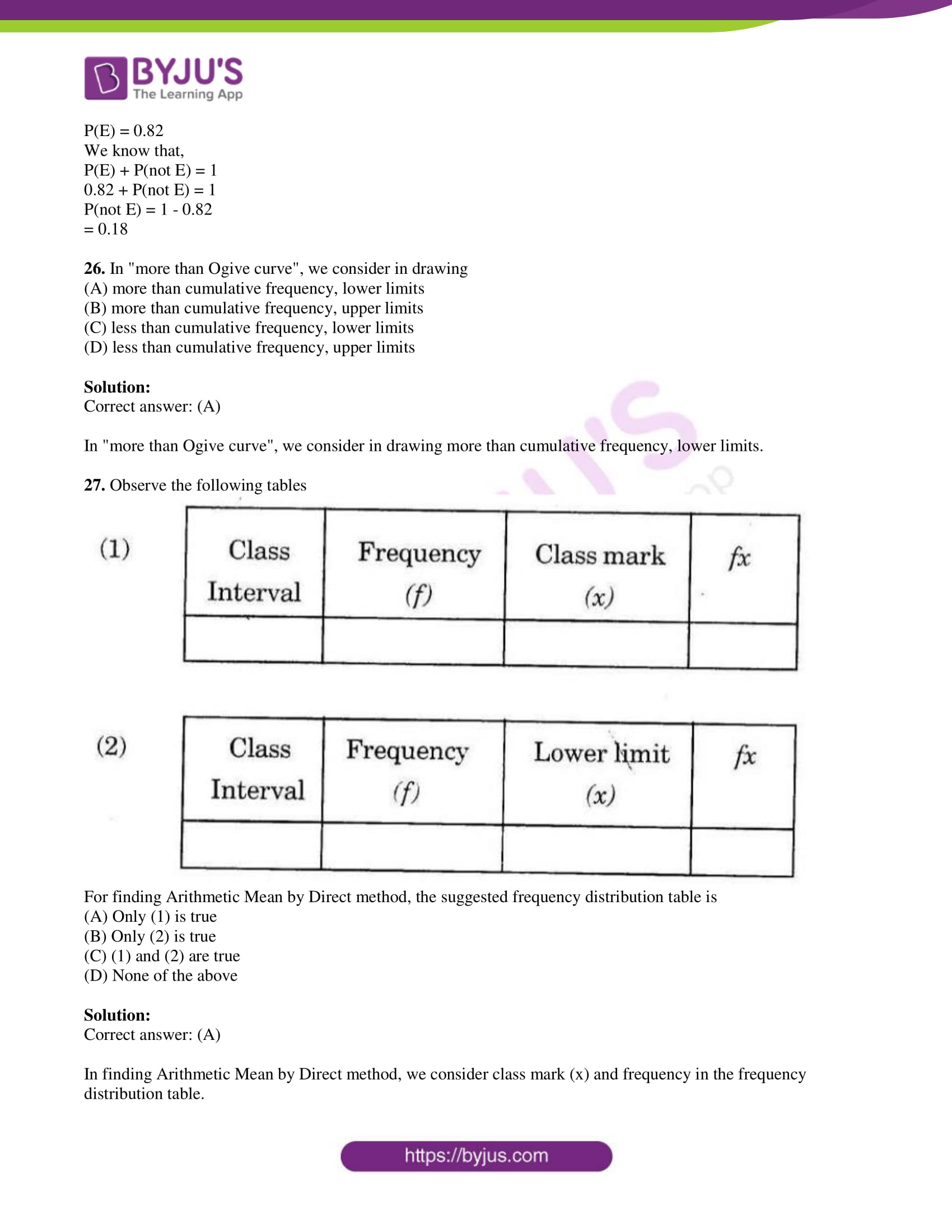PART A

SECTION – I

1. If a cylinder and a cone are of the same radius and height, then how many cones full of milk can fill the cylinder? Answer with reasons.

Solution:

Given,

Height of cylinder = Height of cone = h

Now,

Volume of cylinder = n × Volume of cone

πr2h = n × (1/3) πr2h

⇒ n = 3

Hence, 3 cones full of milk can fill the cylinder.

2. In a ΔDEF, A, B, and C are the midpoints of EF, FD and DE respectively. If the area of ΔDEF is 14.4 cm2, then find the area of ΔABC.

Solution:

Given,

A, B, and C are the midpoints of EF, FD and DE respectively of a ΔDEF.

ar(ΔABC) = (¼) × ar(ΔDEF)

= (¼) × 14.4

= 3.6 cm2

3. When a die is rolled once unbiased, what is the probability of getting a multiple of 3 out of possible outcomes?

Solution:

Total number of outcomes = 6

i.e. {1, 2, 3, 4, 5, 6}

Number of favourable outcomes = 2

i.e. multiples of 3 = {3, 6}

P(getting a multiple of 3) = 2/6 = ⅓

4. Show that tan2θ – (1/cos2θ) = -1.

Solution:

LHS = tan2θ – (1/cos2θ)

= tan2θ – sec2θ

= -(sec2θ – tan2θ)

= -1

= RHS

Therefore, tan2θ – (1/cos2θ) = -1

5. How many tangents can be drawn to a circle from a point on the same circle? Justify your answer.

Solution:

Only one tangent can be drawn to a circle from a point on its circumference.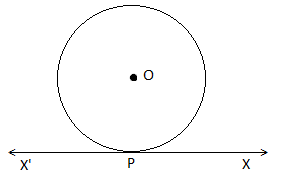XPX’ is the tangent to the circle at a point P.

6.

 Class Interval 10 – 25 25 – 40 40 – 55 55 – 70 70 – 85 85 – 100 Frequency 2 3 7 6 6 6

How do you find the deviation from the assumed mean for the above data?

Solution:

 Class Interval Frequency Midvalues (xi) 10 – 25 2 17.5 25 – 40 3 32.5 40 – 55 7 47.5 = a 55 – 70 6 62.5 70 – 85 6 77.5 85 – 100 6 92.5

Now, deviation = di = xi – A

7. A person from the top of a building of height 25 m has observed another building’s top and bottom at an angle of elevation 45° and at an angle of depression 60° respectively. Draw a diagram for this data.

Solution:

Let CD be the building on which a person observed another building AB.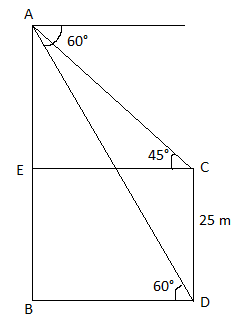SECTION – II

8. A ladder of 3.9 m length is laid against a wall. The distance between the foot of the wall and the ladder is 1.5 m. Find the height at which the ladder touches the wall.

Solution:

Let AB be the wall and AC be the ladder.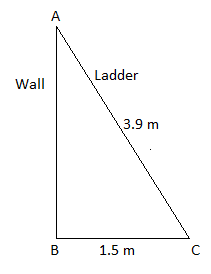In right triangle ABC,

AC2 = AB2 + BC2

AB2 = AC2 – BC2

= (3.9)2 – (1.5)2

= 15.21 – 2.25

= 12.96

AB = 3.6

Therefore, the height at which the ladder touches the wall is 3.6 m.

9. There are 12 red balls, 18 blue balls and 6 white balls in a box. When a ball is drawn at random from the box, what is the probability of not getting a red ball?

Solution:

Given,

12 red balls, 18 blue balls and 6 white balls

Total number of balls = 12 + 18 + 6 = 36

Total number of outcomes = n(S) = 36

Let E be the event of not getting a red ball.

Number of outcomes favourable to E = n(E) = 24

i.e. 36 – 12 (red balls) = 24

P(E) = n(E)/n(S)

= 24/36

= ⅔

Hence, the required probability is ⅔.

10. Prove that “in two concentric circles, a chord of the bigger circle that touches the smaller circle is bisected at the point of contact with the smaller circle”.

Solution:

Let O be the centre of two concentric circles C1 and C2.

Let AB is the chord of the larger circle (C2), which is a tangent to the smaller circle (C1) at P.

Join OP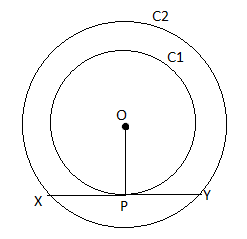OP = Radius of the circle C1

XY = Tangent to the circle C1 at P.

We know that radius perpendicular to the tangent through the point of contact.

OP ⊥ XY

Now, XY is the chord of the larger circle C2 and OP ⊥ XY.

We know that the perpendicular drawn from the centre to the chord always bisects.

Therefore, XD = DY

Hence proved.

11. Show that (1 + cot2θ)(1 – cos θ)(1 + cos θ) = 1

Solution:

LHS = (1 + cot2θ)(1 – cos θ)(1 + cos θ)

Using the identities:

cosec2A – cot2A = 1

sin2A + cos2A = 1

= cosec2θ (1 – cos2θ)

= (1/sin2θ) × sin2θ

= 1

= RHS

Therefore, (1 + cot2θ)(1 – cos θ)(1 + cos θ) = 1

12. The radius of a spherical balloon increases from 7 cm to 14 cm as air is pumped into it. Find the ratio of the volumes of the balloon before and after pumping the air.

Solution:

Given,

Radius of smaller balloon = r = 7 cm

Radius of bigger balloon = R = 14 cm

The volume of sphere = (4/3)πr3

The ratio of volumes = Volume of smaller balloon/ Volume of bigger balloon

= (4/3)πr3/ (4/3)πR3

= r3/R3

= (7 × 7 × 7)/ (14 × 14 × 14)

= 1/8

Hence, the ratio of the volumes of the balloon before and after pumping the air is 1 : 8.

13. Observe the below diagram and find the values of x and y.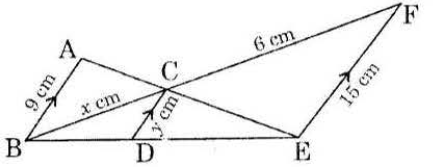Solution:

In ΔABC and ΔEFC,

∠ACB = ∠ECF (vertically opposite angles)

∠A = ∠F (corresponding angles)

By AA similarity,

ΔABC ~ ΔEFC

AB/EF = BC/FC

9/15 = x/6

x = (9 × 6)/15

x = 18/5 = 3.6 cm

CD || EF

BF is the transversal of parallel lines CD and EF

CD/EF = BC/BF

y/15 = x/(x + 6)

y = (3.6/9.6) × 15

y = 5.625 cm

SECTION – III

14. In a village, an enumerator has surveyed for 25 households. The size of the family (number of family members) and the number of families is tabulated as follows.

 Size of the family (No.of members) 1 – 3 3 – 5 5 – 7 7 – 9 9 – 11 No. of families 6 7 9 2 1

Find the mode of the data.

Solution:

 Size of the family (No.of members) No. of families 1 – 3 6 3 – 5 7 = f0 5 – 7 9 = f1 7 – 9 2 = f2 9 – 11 1

Maximum frequency = 9

Modal class = 5 – 7

l = 5

Mode = l + [(f1 – f0)/ (2f1 – f0 – f2)] × h

= 5 + [(9 – 7)/ (2 × 9 – 7 – 2)] × 2

= 5 + (2/9) × 2

= 5 + (4/9)

= 5 + 0.444

= 5.444

OR

There are 100 flash cards labelled from 1 to 100 in a bag. When a card is drawn from the bag at random, what is the probability of getting

(i) a card with prime number from possible outcomes

(ii) a card without prime number from possible outcomes.

Solution:

Total number of outcomes = n(S) = 100

(i) Let E be the event of getting a card with a prime number.

Number of prime numbers from 1 to 100 = 25

Number of outcomes favourable to E = n(E) = 25

P(E) = n(E)/n(S)

= 25/100

= ¼

(ii) P(a card without prime number from possible outcomes) = 1 – P(E)

= 1 – (¼)

= (4 – 1)/4

= ¾

15. Find the value of (sec 15°/ cosec 75°) + (sin 72°/ cos 18°) – (tan 33°/ cot 57°).

Solution:

(sec 15°/ cosec 75°) + (sin 72°/ cos 18°) – (tan 33°/ cot 57°)

= [sec (90° – 75°)/ cosec 75°] + [sin (90° – 18°)/ cos 18°] – [tan (90° – 57°)/ cot 57°]

= (cosec 75°/cosec 75°) + (cos 18°/cos 18°) – (cot 57°/cot 57°)

= 1 + 1 – 1

= 1

OR

Observe the figure given below.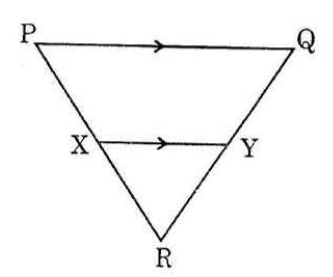In ΔPQR, if XY || PQ, PX/XR = 5/3 and QR = 7.2 cm, then find the length of RY.

Solution:

Given,

XY || PQ, PX/XR = 5/3

PR = PX + XR = 5 + 3 = 8 cm

By BPT,

XR/PR = RY/QR

⅜ = RY/7.2

⇒ RY = (3 × 7.2)/8

⇒ RY = 2.7 cm

16. An observer flying in an aeroplane at an altitude of 900 m observes two ships in front of him, which are in the same direction at an angles of depression of 60° and 30° respectively. Find the distance between the two ships.

Solution:

Let AB be the altitude of aeroplane.

C and D be the positions of two ships.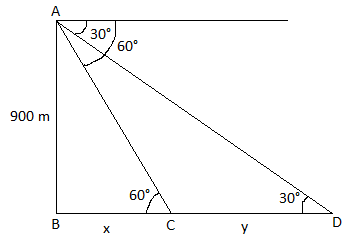In triangle ABC,

tan 60° = AB/BC

√3 = 900/x

x = 900/√3

x = 300√3 m

In triangle ABD,

tan 30° = AB/BD

1/√3 = 900/(x + y)

300√3 + y = 900√3

y = 900√3 – 300√3

y = 600√3 m

Therefore, the distance between two ships is 600√3 m.

OR

A medicine capsule is in the shape of a cylinder with two hemispheres stuck to each of its ends. If the length of the cylindrical part of the capsule is 14 mm and the diameter of hemisphere is 6 mm, then find the volume of medicine capsule.

Solution:

Given that a medicine capsule is in the shape of a cylinder with two hemispheres stuck to each of its ends.

Length of cylindrical part = h = 14 mm

Diameter of hemisphere = 6 mm

Radius of hemisphere = r = 6/2 = 3 mm

Volume of capsule = Volume of cylindrical part + 2(Volume of hemisphere)

= πr2h + 2[(2/3)πr3]

= (22/7) × 3 × 3 × 14 + (4/3) × (22/7) × 3 × 3 × 3

= 396 + 113.14

= 509.14 mm3

17. Draw a circle with radius 3 cm and construct a pair of tangents from a point 8 cm away from the centre.

Solution: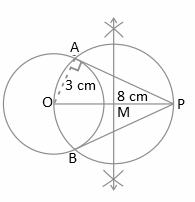PA and PB are the required tangents to the circle.

OR

Daily expenditure of 25 householders is given in the following table:

 Daily expenditure of a household (in Rupees) 100 – 150 150 – 200 200 – 250 250 – 300 300 – 350 No. of households 4 5 12 2 2

Draw a “less than type” cumulative frequency Ogive curve for this data.

Solution:

Less than cumulative frequency distribution table:

 CI cf Less than 150 4 Less than 200 9 Less than 250 21 Less than 300 23 Less than 350 25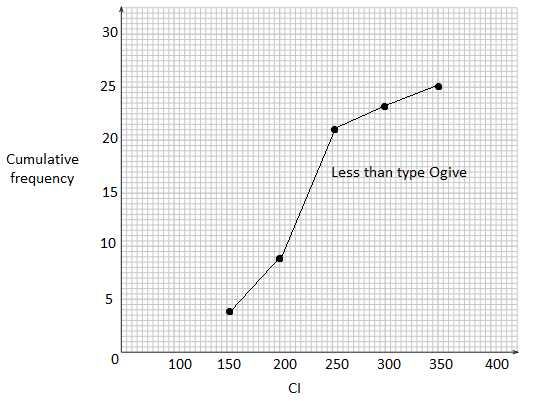SECTION – IV

18. When we construct a triangle similar to a given triangle as per given scale factor, we construct on the basis of

(A) SSS similarity

(B) AAA similarity

(C) Basic proportionality theorem

(D) A and C are correct

Solution:

When we construct a triangle similar to a given triangle as per given scale factor, we construct on the basis of SSS and AAA similarity criterion.

19. If ΔABC ~ ΔEDC, then which of the following representation of figures is true?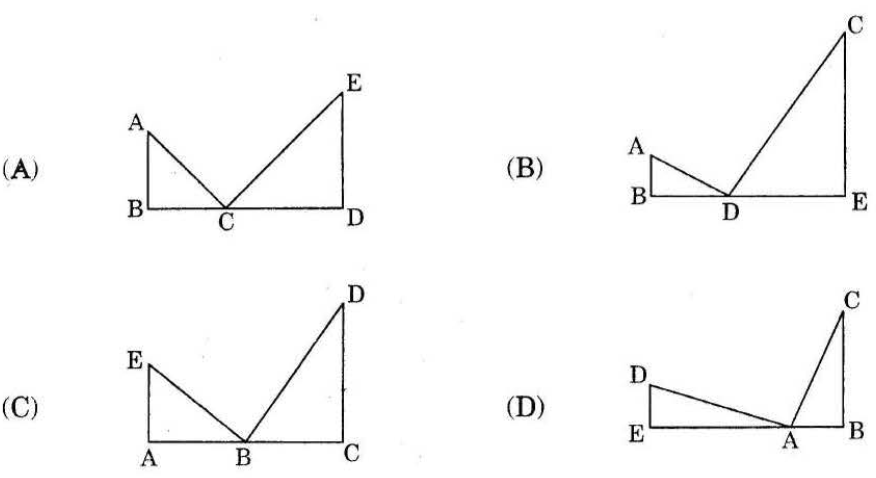Solution:

From the given figures,

ΔABC ~ ΔEDC is true for option (A)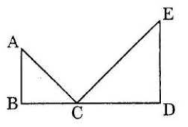20. The number of pair of tangents can be drawn to a circle, which are parallel to each other are

(A) 0

(B) 2

(C) 4

(D) infinite

Solution:

Two tangents can be drawn to a circle, which are parallel to each other, i.e. at endpoints of the diameter of circle.

21. For a right circular cone with radius = r, height = h and slant height = l, which of the following is not true?

(A) Always l > h

(B) Always l > r

(C) Always r > π

(D) l2 = r2 + h2

Solution:

For a right circular cone:

The following are always true.

l > r, l > h

And

l2 = r2 + h2

22. If cot A = 5/12, then sin A + cos A is

(A) 17/13

(B) 12/13

(C) 5/13

(D) 20/13

Solution:

Given,

cot A = 5/12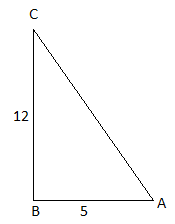By Pythagoras theorem,

AC2 = (12)2 + (5)2

= 144 + 25

= 169

AC = 13

sin A + cos A = (12/13) + (5/13)

= 17/13

23. Which of the following values is not a possible value of sin x?

(A) 3/4

(B) 3/5

(C) 4/5

(D) 5/4

Solution:

We know that,

0 ≤ sin x ≤ 1

¾ < 1

⅗ < 1

⅘ < 1

5/4 > 1

Therefore, 5/4 is not a possible value of sin x.

24. A ladder ‘x’ meters long is laid against a wall making an angle θ with the ground. If we want to directly find the distance between the foot of the ladder and the foot of the wall, which triogonometrical ratio should be considered?

(A) sin θ

(B) cos θ

(C) tan θ

(D) cot θ

Solution:

Let AB be the wall and AC be the ladder.

BC is distance between the foot of the ladder and the foot of the wall.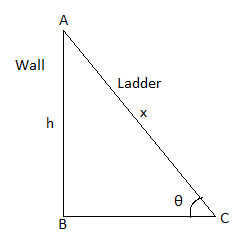cos θ = BC/AC

25. If P(E) = 0.82, then P(not E) =

(A) 0.18

(B) 0.28

(C) 0.38

(D) P(E) = P(not E)

Solution:

Given,

P(E) = 0.82

We know that,

P(E) + P(not E) = 1

0.82 + P(not E) = 1

P(not E) = 1 – 0.82

= 0.18

26. In “more than Ogive curve”, we consider in drawing

(A) more than cumulative frequency, lower limits

(B) more than cumulative frequency, upper limits

(C) less than cumulative frequency, lower limits

(D) less than cumulative frequency, upper limits

Solution:

In “more than Ogive curve”, we consider in drawing more than cumulative frequency, lower limits.

27. Observe the following tables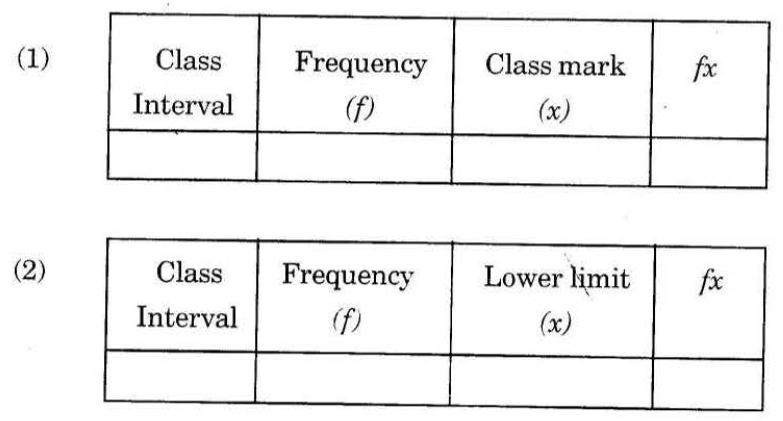For finding Arithmetic Mean by Direct method, the suggested frequency distribution table is

(A) Only (1) is true

(B) Only (2) is true

(C) (1) and (2) are true

(D) None of the above

Solution: